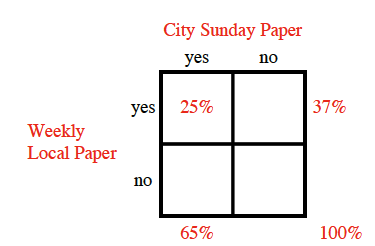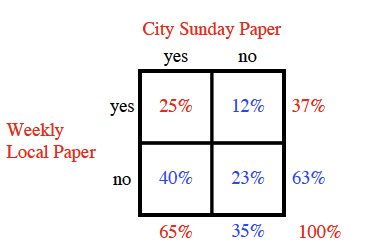Home > CCAA8 > Chapter cca10 > Lesson cca10.1.1 > Problem10-12

10-12.

In a certain small town, 65% of the residents subscribe to the city Sunday paper, 37% subscribe to the weekly local paper, and 25% subscribe to both papers. Homework Help ✎

1. What are the two variables? Create a two-way table.2. If a resident is selected at random, what is the probability he or she subscribes to at least one of the two papers?

Which three boxes in the two-way table represent someone subscribing to at least one paper?

$25\%+12\%+40\%=77\%$

1. Charlie’s neighbor subscribes to a paper. What is the probability that he takes the Sunday paper?

• This is the probability of someone subscribing to the Sunday paper given the information
that they subscribe to at least one paper. Use your answer from part (b).

$\frac{65}{77}=?$